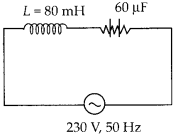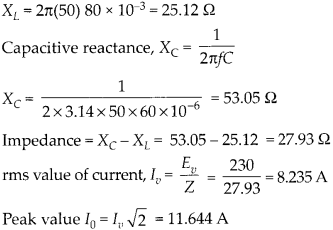Enlightened

# Question 18: NCERT Solutions for 12th Class Physics: Chapter 7-Alternating Current

• 0

Question 18: NCERT Solutions for 12th Class Physics: Chapter 7-Alternating Current

A circuit containing a 80 mH inductor and a 60 μF capacitor in series is connected to a 230 V, 50 Hz supply. The resistance of the circuit is negligible.
(a) Obtain the current amplitude and rms values.
(b) Obtain the rms values of potential drops across each element.
(c) What is the average power transferred to the inductor?
(d) What is the average power transferred to the capacitor.
(e) What is the total average power absorbed by the circuit? [‘Average’implies’averaged over one cycle’.

Share

1. Solution:(a) Inductive reactance , XL = 2πƒL(b) Potential drop across L, VL = lυXL   X

(c) Average power transferred to inductor is zero, because of phase difference π/2(d) Average power transferred to capacitor is also zero, because of phase difference π/2(e) total power absorbed by the circuitCheck the complete chapter with solutions.

NCERT Solutions for 12th Class Physics: Chapter 7-Alternating Current

• 0